# Protection of MTDC Network Using a Resistive Type Superconducting Fault Current Limiter

Protection of MTDC Network Using a Resistive Type Superconducting Fault Current Limiter
Laboratoire de Maitrise des Energies Renouvelables (LMER), Faculté de Technologie, Université de Bejaia, Bejaia 06000, Algeria

Groupe de Recherche en Energie Electrique de Nancy (GREEN), Faculté des Sciences et Technologies Université de Lorraine, Vandoeuvre-lès-Nancy 54506, France

Corresponding Author Email:
yacineayachiamor@gmail.com
Page:
475-480
|
DOI:
https://doi.org/10.18280/ejee.230607
6 January 2020
|
Accepted:
10 December 2021
|
Published:
31 December 2021
| Citation

OPEN ACCESS

Abstract:

Multi-terminal DC network (MTDC) offers great potential for long distance huge power delivery with multi-direction power transmission capability. However, the key obstacle in a realization of MTDC is the lack of existing commercial protection device can withstand the DC fault that rises rapidly and surge tenfold within several milliseconds over the whole system. The new technology called Superconducting Fault Current Limiter (SFCL) could bring a solution to the main bottleneck of the MTDC networks. In this work, an electro-thermal model of resistive type SFCL in series with a hybrid DC circuit breaker is proposed to protect a five terminal MTDC network. The numerical analysis carried out using (EMTP-RV®) software, and the simulation results show how effectively the SFCL can reduce the fault current and increase the breaking capability. Moreover, system stability is remarkably improved.

Keywords:

five terminal MTDC, resistive type SFCL, hybrid DC circuit breaker, DC fault, transient stability

1. Introduction

The significant increasing of energy demand as well the greenhouse emission leads to change the energy pattern with more climate-friendly energy resources such as hydro, wind or solar. Therefore, it is essential to accommodate these renewable energies by using a cost-effective transmission network. Multiterminal HVDC (MTDC) grids are seen as the main solution for the increasing electricity demand and integration of largescale renewable energies for long distance bulk power delivery . MTDC system has advantages over conventional two terminal point-to-point HVDC system in many aspects such as control flexibility, reliability and economy .

However, the DC fault current in MTDC system rises much more rapidly and has larger magnitude (tens of kilo Amps) compared with the point-to-point HVDC systems due to multiple power source linked to the terminals of MTDC. At present, using circuit breakers to cut off the fault current is widely applied in the DC system to ensure the whole system safety. However, the rated ultimate breaking capacity is limited. Recently, the DC breaker developed by ABB can cut off a 16 kA fault current within 2 ms while its rated voltage is 320 kV , and Alstom has developed a dc breaker that can cut off a 7.5 kA fault current within 1.6 ms while the rated voltage is 120 kV .

The Superconducting Fault Current Limiter (SFCL) technology could bring a solution to the main bottleneck of the MTDC networks as well as to the fault current interruption by offering a huge reduction in the DC fault and reduce the stress across the circuit breaker. Not limited to that, but it increases the system stability and distributed energy quality also [5, 6]. The operation principal of the proposed SFCL is based on the electro-thermal behavior. When the passing current exceeds the critical current and the temperature exceeds the critical value, the SFCL’s impedance increases exponentially to the maximum impedance level what’s called quenching process, this phenomenon happens in a very short time called transition time called quenching speed.

Various design technology of SFCL has been considered, resistive SFCL, saturable-core SFCL, shielded-core SFCL and non-superconducting technologies . For HVDC systems, the resistive SFCL is commonly designed due to its simplicity and reliability. The application of SFCL in different DC grid systems have been investigated, including DC grids protected by slow mechanical DCCBs [8, 9], point-to-point HVDC [7, 10] and radial three-terminal MTDC grid . In this work, the second-generation high-temperature superconductor 2G HTS r-SFCL has been accurately modelled using ElectroMagnetic Transient Program (EMTP-RV®) software considering the electro-thermal behavior. The proposed r-SFCL is working together with fast protection hybrid DC breaker (HCB) to protect a meshed five terminal MTDC grid against a worst DC fault scenario.

The structure of this paper is as follows: Section 2 presents the complete architecture of the proposed five terminal MTDC grid. Section 3 lunches the detailed model of the line protection that composed of r-SFCL and HCB. While section 4 illustrates the simulation results and discussion. Finally, the paper end-up with conclusion in section 5.

2. Five Terminal MTDC Network

MTDC system is simply an extension of point-to-point HVDC (more than two terminals). In this work, a five terminal MTDC mesh grid with bipolar topology will be adopted to perform the analysis, Figure 1 shows the proposed system including all the parameters. The architecture of this grid is inspired by European TWENTIES project . The system consists of 5 Modular Multilevel Converter (MMC) each one made up of 400 half-bridge sub-modules (SMs) per arm. MMC 3, 4 and 5 are controlled based on power control whereas MMC 1 and 2 are controlled by DC voltage droop control with droop value K=0.82 pu. Where K=ΔVdc/ΔP.

The HVDC cable is based on bipolar underground transmission cables with a wideband modelling approach where the cable data taken from France-Spain HVDC link (the model is available in EMTP-RV®) .

## 1.png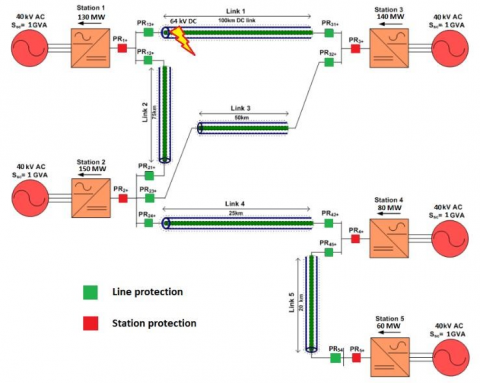Figure 1. Five terminal MTDC grid

3. Line Protection Scheme

Since the fault is applied on the level of the cables, only line protections will be considered in this study, where the r-SFCL is supposed to be placed between the hybrid DC circuit breaker and the DC bus terminal as shown in Figure 2. The details of the system model are explained in the following.

## 2.png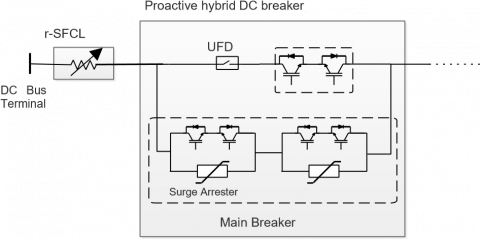Figure 2. Resistive type SFCL in series with hybrid DC circuit breaker

3.1 The electro-thermal model of r-SCFL

The r-SFCL model used in simulations is based on HTS coated conductor most often called HTS tape. These tapes are based on the architecture illustrated in Figure 3, i.e. a stack of buffer layer (oxides), and a superconducting layer, e.g. (RE)BCO, deposited on a mechanically strong metallic substrate (stainless steel and Hastelloy). The model can be decomposed into two parts, an electrical part and a thermal part in which they are coupled to obtain a valid r-SFCL model.

## 3.png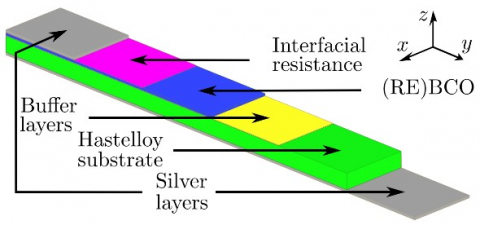Figure 3. Example of HTS tape architecture implemented in the MTDC network

3.1.1 Electrical model

Each layer of the HTS tape can be modeled by a nonlinear resistance that depends of current I and/or temperature T,

$R_{e l}(J, T)=\rho(J, T) \frac{L}{A}$     (1)

where, $\rho(J, T)$  is the resistivity of the considered material ((RE)BCO, silver or Hastelloy), L is the length of the tape and A is its longitudinal cross-section. The current density J is given by $J=I / A$  where I represents the total current flowing through Rel. Except for the superconducting tape, the properties of all materials constituting HTS tape depend only on T [13, 14].

The resistivity of the superconducting layer is calculated by the well-known power law characteristics at law electrical field,

i.e.

$\rho(J, T)=\frac{E_{c}}{J_{c}(T)}\left(\frac{|J|}{J_{c}(T)}\right)^{n-1}$     (2)

where, Ec is the electrical field criterion used to define the critical current density of the (RE)BCO superconductor Jc, and n is the power law exponent.

The temperature dependence of Jc can be evaluated by:

$J_{c 0}(T)=J_{c 0}\left(\frac{T_{c}-T}{T_{c}-T_{0}}\right)$     (3)

where, T0 is the liquid temperature of nitrogen bath, Tc is the critical temperature of the (RE)BCO superconductor, and Jc0 is the critical current density in self-magnetic field at T=T0. The material parameters used for the superconductor are given in Table 1.

Table 1. Parameters of the (RE)BCO superconductor

 Parameters Value Description $E c$ 1 $\mu \mathrm{V}$ cm-1 Critical electrical field criterion $J c 0$ 2.5 MA cm-2 Self-field critical current density n 15  Power law exponent a 0.47 $\mu \Omega$ cm-1  Temperature coefficient Tc 90 K Critical temperature T0 77 K Temperature of the LN2 bath

3.1.2 Thermal model

Take into account the evolution of the temperature during the transition of the tape is critical for achieving reliable simulations. As the model is developed in a power system environment (EMTP-RV), the simplest approach is to express the thermal model in terms of electric circuit elements by the use of “thermal to electrical” analogy .

To evaluate this mean temperature, the Joules losses Qj in each layer j are computed by

$Q j(I, T)=\operatorname{Rel}(I j, T) \cdot I j 2$     (4)

The general thermal equation can be rewritten as a lumped model with average temperature T and heat capacity Cth by the use of the following equation:

$C_{t h}(T)=\frac{d T}{d t}=Q-Q_{c}=\sum_{j=1}^{n} Q_{j}\left(i_{j}, T\right)-Q_{c}(\Delta T)$     (5)

where, Q-Qc is the total internal heat gains minus the heat evacuated through convection noted Qc. The cooling power Qc is evaluated by the use of the nonlinear Newton law $Q c=h(T s-T 0) S(T s-T 0)$  where TS is the surface temperature of the tape (equal to the mean temperature T in this model), T0 is the cryogenic bath temperature considered equal to 77K (LN2 bath), S is the transverse cross section and $h(T s-T 0)$ is an effective nonlinear steady state coefficient, expressed in W.K1m-2. With Eqns. (4) and (5), the evolution of the temperature T(t) can be computed for a given current I(t) imposed in the r-SFCL.

3.2 Hybrid DC circuit breaker modeling

The hybrid DC circuit breaker (HCB) is innovated and developed by ABB . Figure 2 shows the structure of the HCB. This circuit breaker is a combination of mechanical switches and semiconductor switches. It is shown that the circuit breaker has two parallel branches: The primary branch consists of an Ultra-Fast Disconnector (UFD) in series with a group of semi-conductor switches known as the Load Commutation Switch (LCS). The secondary branch is the main DC breaker, consisting of a string of semiconductor switches connected in parallel groups with a large bank of varistors that used to limit the transient recovery voltage (TRV).

During normal operation, LCS and UFD are closed and conducting whereas the main breaker is open. When the fault is detected, the LCS is turned off and the LCS’s snubber circuit charges  and produces a voltage to commutate the current from the primary branch into the main breaker. Once the current is fully commutated to the main breaker, the UFD will open effortlessly with minimum arc. Now, when the main breaker is switched off to break the fault current, a TRV appears across the circuit breaker, which is limited by the surge arresters. This latter must be rated to deal with the peak of TRV.

Compared with the conventional structure, some modifications have been inserted, where the inductor that used to be placed in series with any hybrid HVDC circuit breaker to limit the fault current rising is completely removed and replaced by the proposed r-SFCL. Secondly, all the commutation switches stage are modified into a bi-directional current breaking model by adding mirror pair of IGBT valves, so that the breaker has the ability to break the current in both directions .

4. Simulation Results

In order to validate the performance of the proposed protection scheme, a pole-to-pole fault DC fault with a low impudence equals to 0.1 Ω at the endpoint of link 1 close to station 1 is imposed (see Figure 1). This type of fault considered as the worst scenario case that could happen to the grid, the fault appears at t=1 s and lasts after 100 ms. Two cases of analysis will be discussed in this work, the first one will assess the impact of the r-SFCL on the grid without any breaking functionality, whereas the second case, the HCB is inserted to the grid, working together with the r-SFCL to cutoff the faulty current in very fast manner and with low breaking capability.

4.1 The impact of the r-SFCL on the grid without any breaking

Figures 4, 5, 6, 7 and 8 illustrate the stations current. As depicted in Figure 4, the peak fault current can reach up to approximately 39 kA. With r-SFCL applied on both ends of the transmission line, it is shown that the current peak is limited to about 12 kA. In other word, the fault current is limited up to 70% from the fault condition without r-SFCL. In addition, the SFCL enhance the margin stability after the fault disappearance, where it is noticed that the current has low undershot and reach the steady state faster. It is noticed also that as the stations get far from the fault location as the peak fault current corresponding to that station is lower.

From the stability point of view, the transient stability in AC grids refers to the ability of a power system to maintain synchronism when subjected to severe disturbances. Similarly, in DC grids, transient stability can be defined as the ability of the DC grid to maintain DC voltage level when the system is subjected to large-scale disturbances such as DC faults .

## 4.png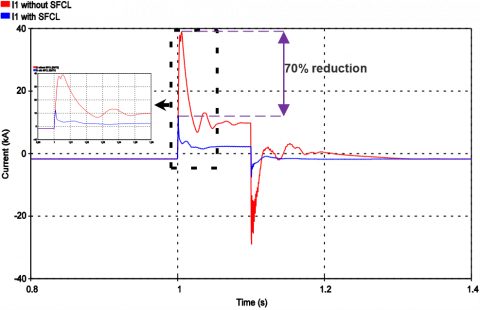Figure 4. Station 1 current

## 5.png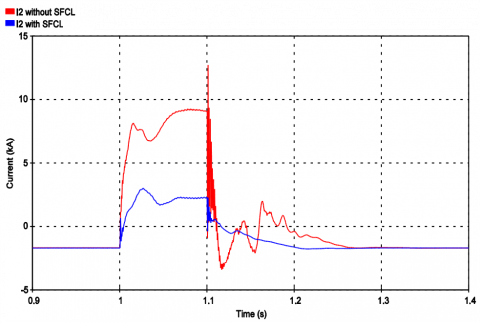Figure 5. Station 2 current

## 6.png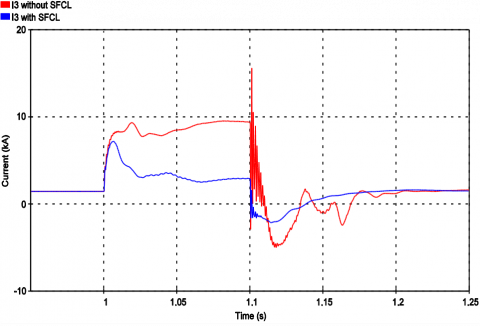Figure 6. Station 3 current

## 7.png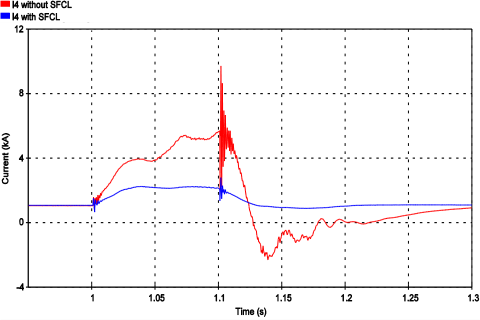Figure 7. Station 4 current

## 8.png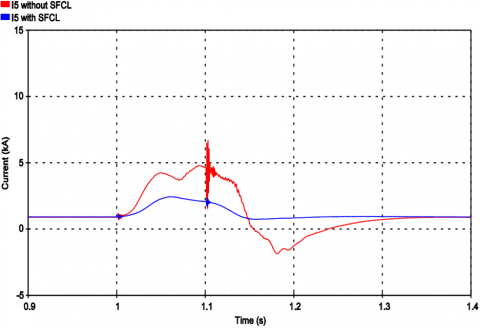Figure 8. Station 5 current

## 9.png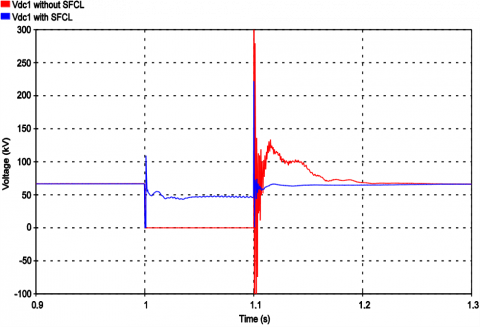Figure 9. Station 1 voltage

Figure 9 demonstrates the station 1 voltage drop, it is clearly shows that r-SFCL prevent the station form losing the control during the fault by elevating the voltage drop from almost 0 kV to 48 kV, knowing that the loss of controllability when the voltage is below 80% of the nominal voltage according to reference .

The voltage drop in the other stations depends mainly on the electrical distance from the fault location and the equivalent station’s reactors, which implies that all other station’s voltage kept above the voltage drop of the first station.

4.2 Fault current clearing using r-SFCL and HCB

In order to cut off the fault current as fast as possible with minimum breaking capability, the hybrid DC breaker (HCB) has been put in series with r-SFCL. Assuming the selectivity function is provided, that means only line 1 will be interrupted. Figure 10 shows that the current is successfully interrupted within 5 ms, while the breaking current capability kept below the limitation of the HCB when the r-SFCL is applied.

Figure 11 assesses the power flow behavior before, during and after the fault interruption. (Given that: a positive power is when the power flows from the converter to the DC line). Since only the faulty line is interrupted, the continuity of the power flow is guaranteed during the fault time between 1 s and 1.1 s. After the fault disappearance, the HCB reclose automatically and the power flow recovers its initial rated value.

## 10.png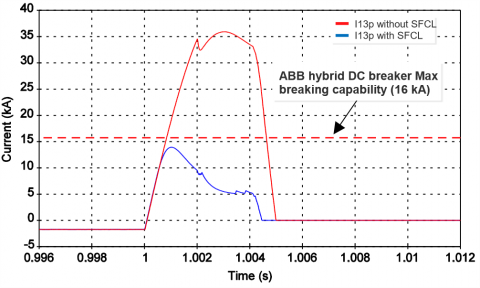Figure 10. Positive pole line current I13

## 11.png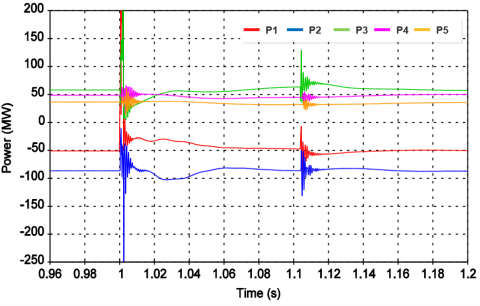Figure 11. Power flow before, during, and after the fault

4.3 The thermal behavior of the r-SFCL

## 12.png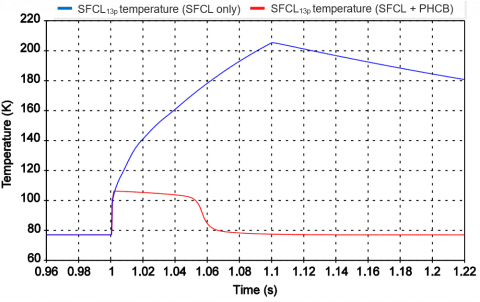Figure 12. SFCL13p thermal behaviour

The thermal behavior of SFCLs located on the positive pole of station 1 (SFCL13p) was measured assuming homogeneous quenching of the tape. The test was carried out with and without HCB. It is noticed in Figure 12 that for all cases the maximum admissible temperature (250 K) is respected.

For the case where the HCB is not applied (blue curve), the r-SFCL quench almost instantaneously when the fault occurs and the temperature starts to increase until reaching 206 K at 1.1 s the time when the fault disappears, then the SFCL13p start to recovery, for this case, the SFCL recovery is not seen as it has a much longer time constant.

When the HCB is applied, the maximum temperature of SFCL13p reaches 107 k when the fault cleared at 4.5 ms (red curve), then the temperature recovers in 67 ms.

5. Conclusions

In this work, a novel approach of a resistive type superconducting fault current limiter with electro-thermal behavior is applied in a five-terminal meshed MTDC grid. The proposed r-SFCL is associated with a fast protection hybrid DC breaker to cut-off the fault current in a very short time with minimum breaking capability. The analysis was validated with a time-domain simulation using EMTP software. The results showed that the fault current could be reduced up to 70% while the system stability margin is dramatically improved. In sum, the DC resistive SFCL is proved to be a very promising technology in multi-terminal HVDC systems under any severe disturbance such as DC fault current.

Acknowledgment

This work was supported by the GDR SEEDS.

References

 Bahrman, M.P. (2006). Overview of HVDC transmission. 2006 IEEE PES Power Systems Conference and Exposition, pp. 18-23. https://doi.org/10.1109/PSCE.2006.296221

 Chen, H.R., Zhang, F., Chang, Y (2006). Improvement of power quality by VSC based multiterminal HVDC. IEEE Power Engineering Society General Meeting. https://doi.org/10.1109/PES.2006.1709257

 Callavik, M., Blomberg, A. (2012). The Hybrid HVDC Breaker An innovation breakthrough enabling reliable HVDC grids. ABB Grid Systems, Technical Paper.

 Chen, Y.X. (2017) Review of the development of multi-terminal HVDC and DC power grid. IOP Conference Series Earth and Environmental Science, 93(1): 012044. https://doi.org/10.1088/1755-1315/93/1/012044

 Sung, B.C., Park, D.K., Ko, T.K. (2009). Study on a series resistive SFCL to improve power system transient stability: Modeling, simulation, and experimental verification. IEEE Transactions on Industrial Electronics, 56(7): 2412-2419. https://doi.org/10.1109/TIE.2009.2018432

 Huang, G.B., Douine, B., Berger, K., Didier, G., Isabelle, S., Lévêque, G. (2016). Increase of stability margin in embedded DC electric grid with superconducting stabilizer. IEEE Transactions on Applied Superconductivity, 26(4): 1-4. https://doi.org/10.1109/TASC.2016.2543963

 Lee, H.Y., Asif, M., Park, K.H., Lee, B.W. (2018). Feasible application study of several types of superconducting fault current limiters in HVDC grids. IEEE Transactions on Applied Superconductivity, 28(4): 1-5. https://doi.org/10.1109/TASC.2018.2799745

 Yang, Q.Q., Le Blond, S., Liang, F., Yuan, W.J., Zhang, M., Li, J.W. (2017) Design and application of superconducting fault current limiter in a multiterminal HVDC system. IEEE Transactions on Applied Superconductivity, 27(4): 1-5. https://doi.org/10.1109/TASC.2017.2669152

 Garcia, W.R.L., Bertinato, A., Tixador, P., Raison, B., Luscan, B. (2016). Full-selective protection strategy for MTDC grids based on R-type superconducting FCLs and mechanical DC circuit breakers. In 5th IET International Conference on Renewable Power Generation (RPG), pp. 1-7. https://doi.org/10.1049/cp.2016.0564

 Zhang, L., Shi, J., Wang, Z., et al. (2017). Application of a novel superconducting fault current limiter in a VSC-HVDC system. IEEE Transactions on Applied Superconductivity, 27(4): 1-6. https://doi.org/10.1109/TASC.2017.2656634

 TWENTIES Project. [Online]. Available: https://windeurope.org/about-wind/reports/twenties-project/.

 Garcia, W.R.L., Bertinato, A., Tixador, P., Raison, B., Luscan, B. (2018). Protection strategy for multi-terminal high- voltage direct current grids using SFCLs at converter station output. The 14th International Conference on Developments in Power System Protection (DPSP 2018), 2018(15): 1369-1374. https://doi.org/10.1049/joe.2018.0224

 Roy, F. (2010). Modeling and characterization of coated conductors applied to the design of superconducting fault current limiters. PhD dissertation EPFL, Lausanne. https://doi.org/10.5075/epfl-thesis-4721

 Ekin, J. (2006). Experimental Techniques for Low Temperature Measurements. Oxford University Press. Oxford. UK.

 Lacroix, C., Sirois, F., Slimani, K., Cave, J.R. (2013). Electro-thermal response of 2G HTS coated conductors subjected to current pulses. IEEE Trans. on Appl. Superconductivity, 23(3): 6601605. https://doi.org/10.1109/TASC.2013.2238285

 Friedmann, T.A., Rabin, M.W., Giapintzakis, J., Rice, J.P., Ginsberg, M. (1990). Direct measurement of the anisotropy of the resistivity in the a-b plane of twin-free, single-crystal, superconducting YBa2Cu3O7−delta. Phys Rev B Condens Matter., 42(10): 6217-6221. https://doi.org/10.1103/physrevb.42.6217

 Bonnard, C.H., Sirois, F., Lacroix, C., Didier, G. (2017). Multi-scale model of resistive-type superconducting fault current limiters based on 2G HTS coated conductors. Superconductor Science and Technology, 30(1): 014005. https://doi.org/10.1088/0953-2048/30/1/014005

 Lin, W., Jovcic, D., Nguefeu, S., Saad, H. (2016). Modelling of high-power hybrid DC circuit breaker for grid-level studies. IET Power Electronics, 9(2): 237-246. https://doi.org/10.1049/iet-pel.2015.0518

 Khan, U.A., Lee, J., Amir, F., Lee, B. (2015). A novel model of HVDC hybrid-type superconducting circuit breaker and its performance analysis for limiting and breaking DC fault currents. IEEE Transactions on Applied Superconductivity, 25(6): 1-9. https://doi.org/10.1109/TASC.2015.2477373

 Pierre, R. (2014). Dynamic modeling and control of multi-terminal HVDC grids. PhD thesis, PRES Université Lille Nord-de-France.

 Endegnanew, A.G., Uhlen, K., Haileselassie, T.M., Anaya-Lara, O. (2016). Transient stability analysis in multi-terminal VSC-HVDC grids. In: 19th Power Systems Computation Conference (PSCC), pp. 1-7. https://doi.org/10.1109/PSCC.2016.7540857